# Inter maths solution for De Moivre’s theorem

Mathematics intermediate second year maths IIA solutions for some problems. These are very easy to understand.

Inter maths solutions for first year 1a and 1b for examination purpose. Inter maths 2a solutions for complex numbers and de moivre’s theorem.

Inter first year 1a and 1b solutions are also available here.

3. Matrices

You can also see the solutions for 1b

1. Locus

The straight line sa

Straight lines la

12. Rate measure

You can see the solutions for maths IIA inter

Complex numbers solutions for textbook

De Mouvre’s Theorem textbook solutions

Exercise 3(b)

Exercise 3(c)

You can see solutions for Inter maths IIB

1. Circle

3. Parabola

4. Ellipse

# De Moivre’s theorem solutions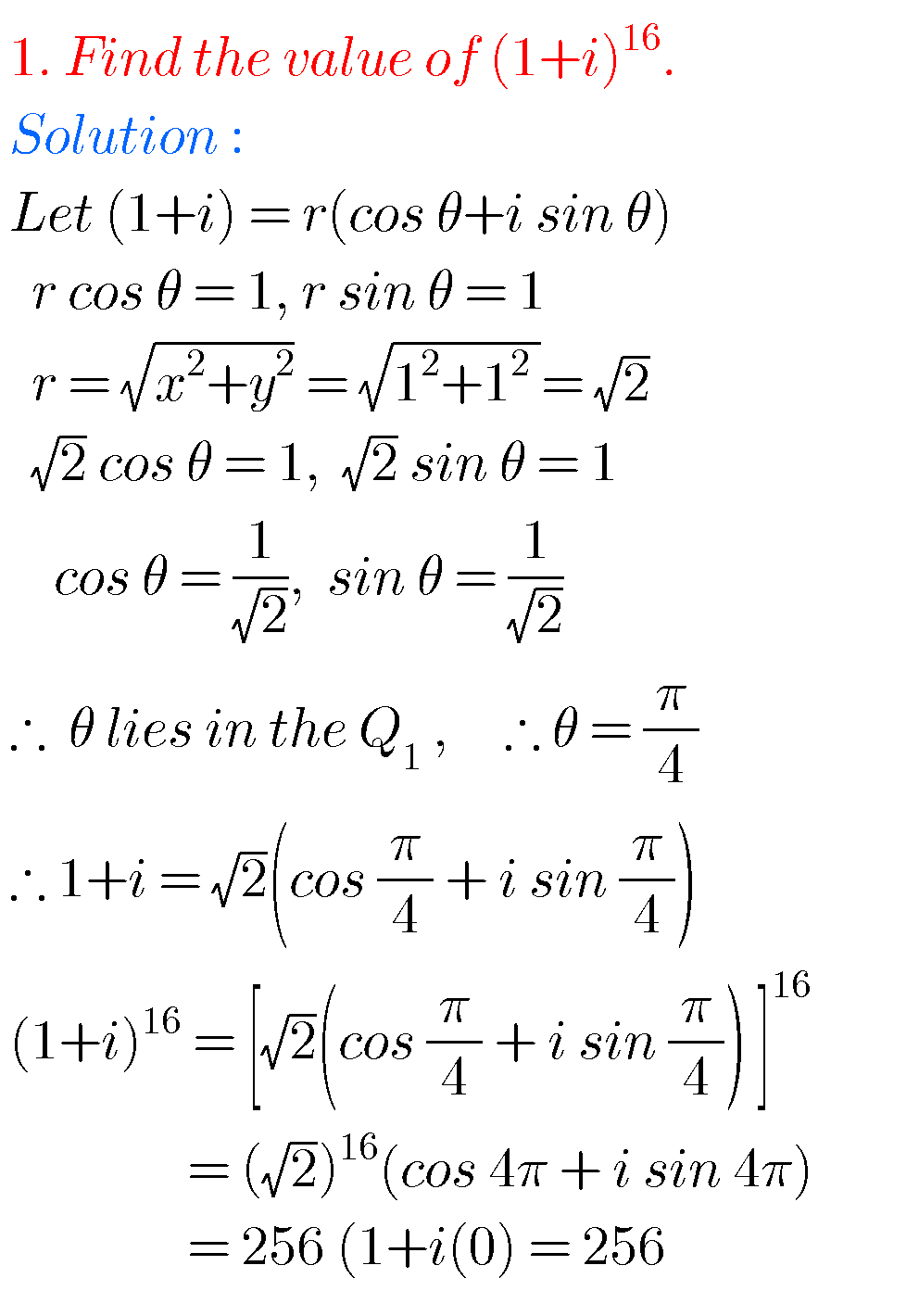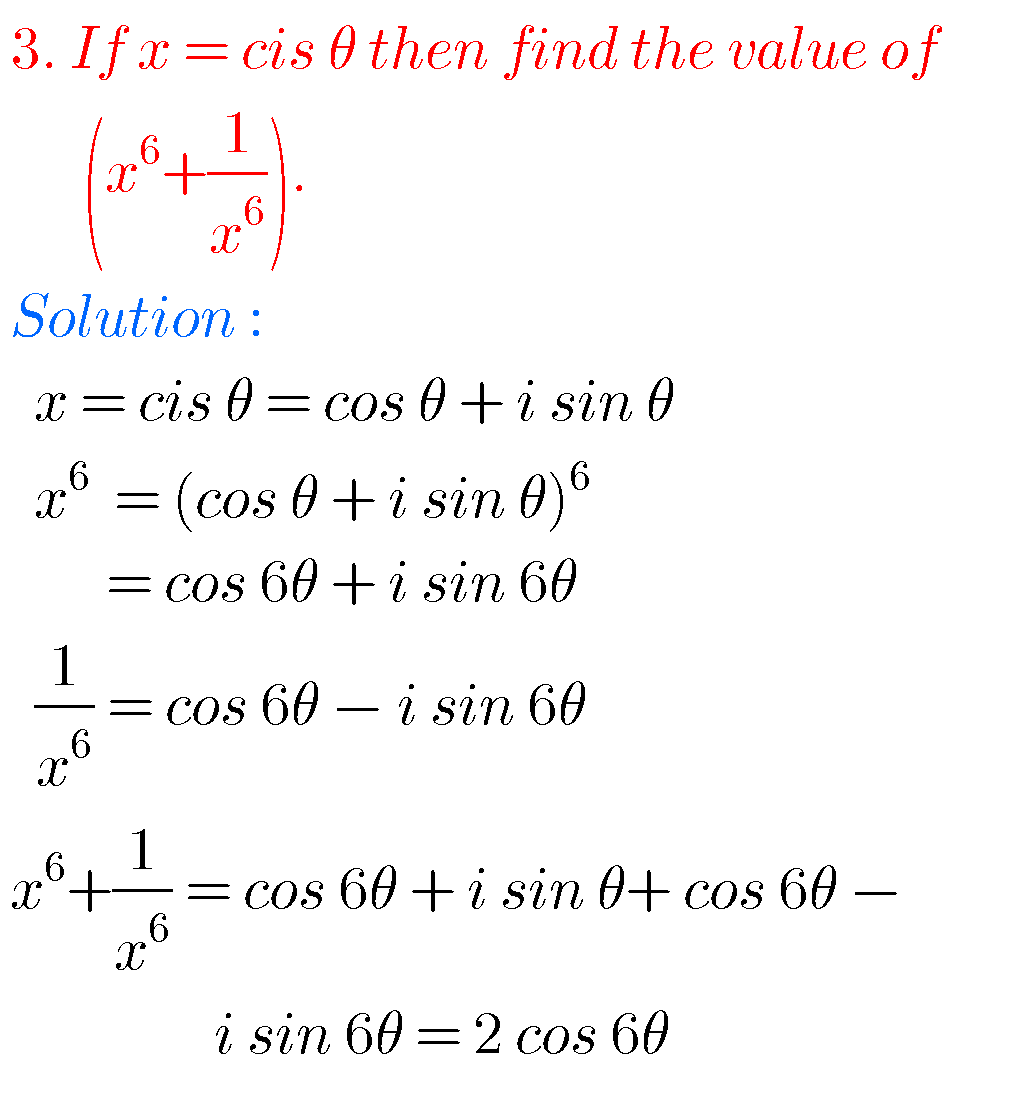##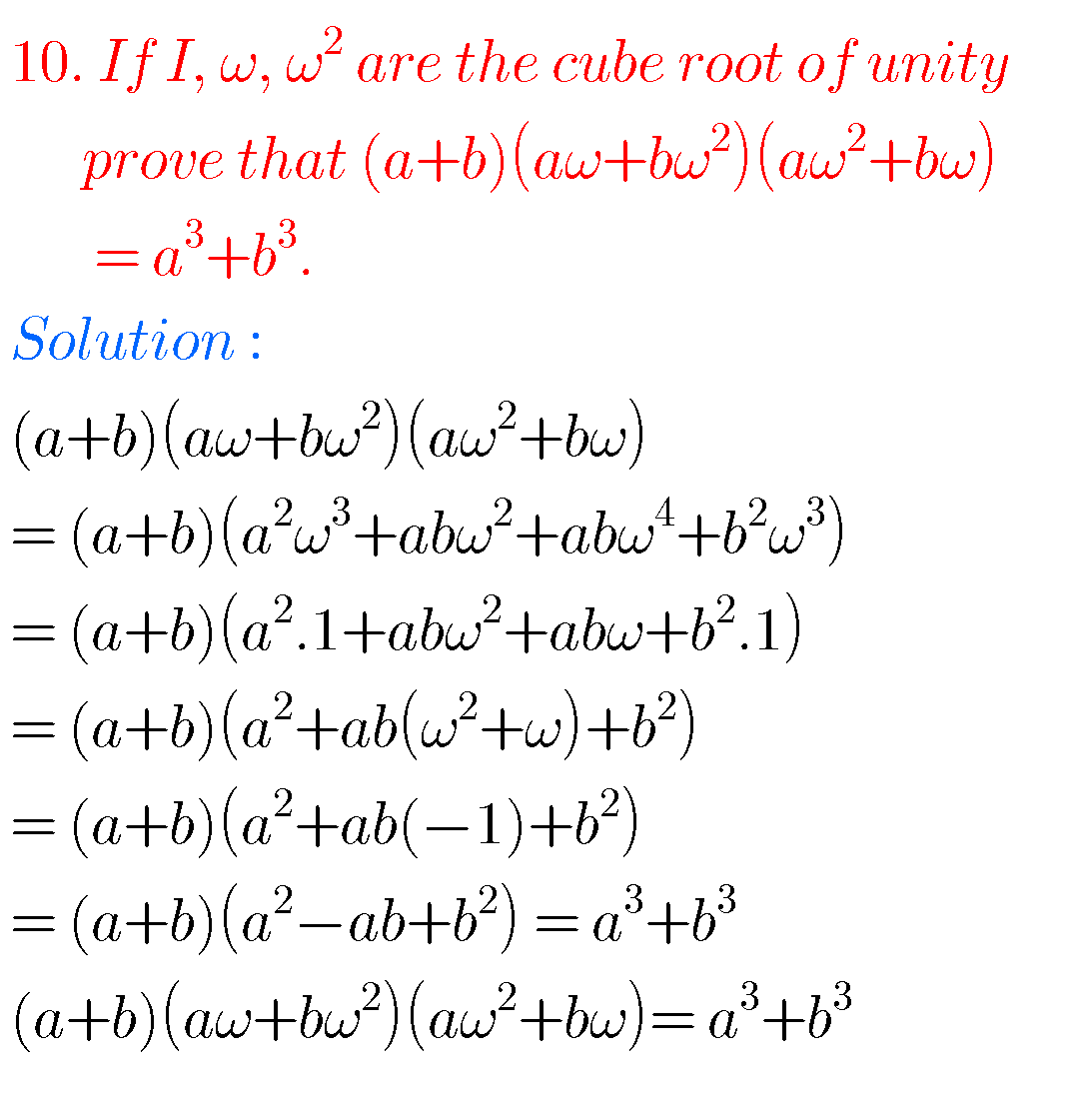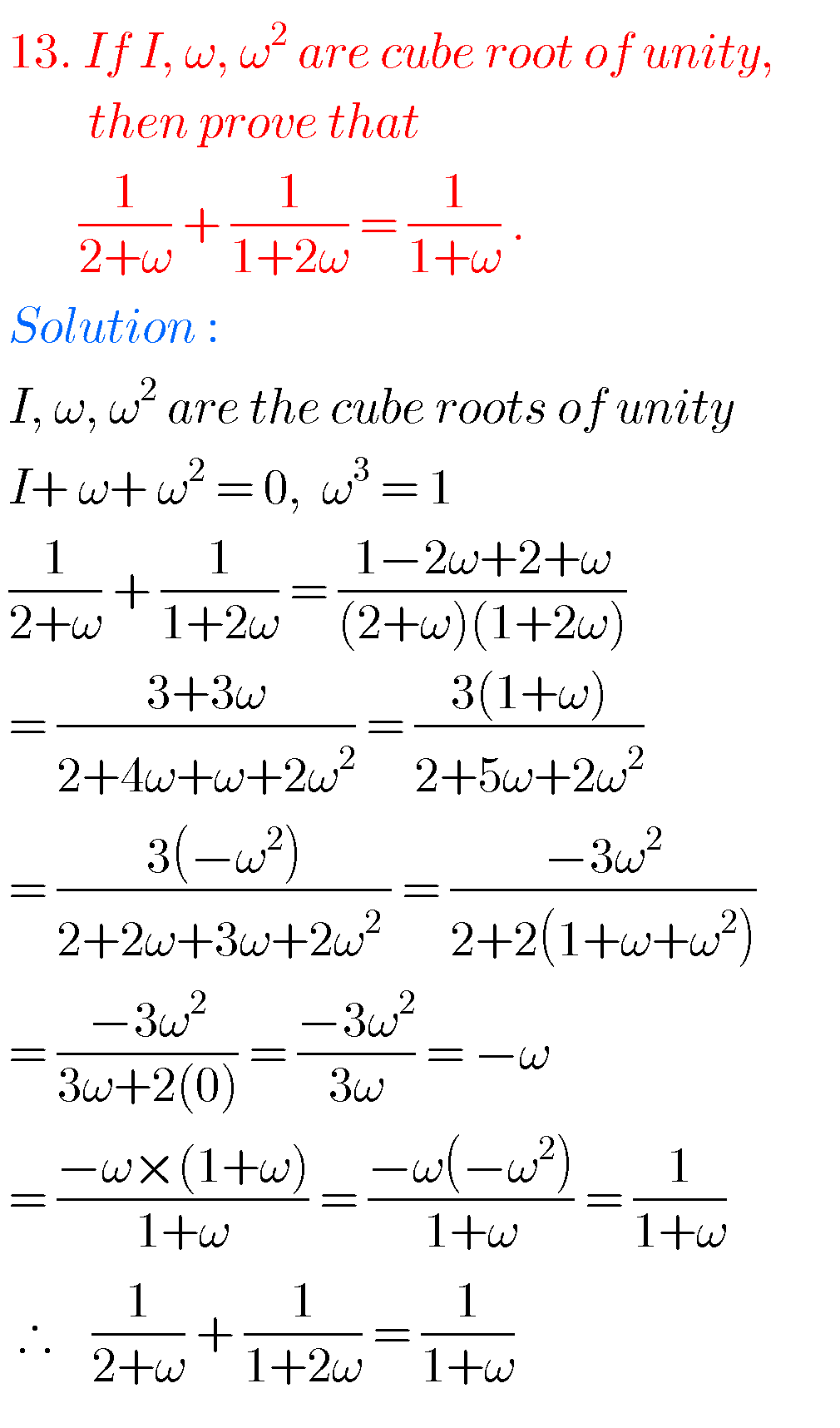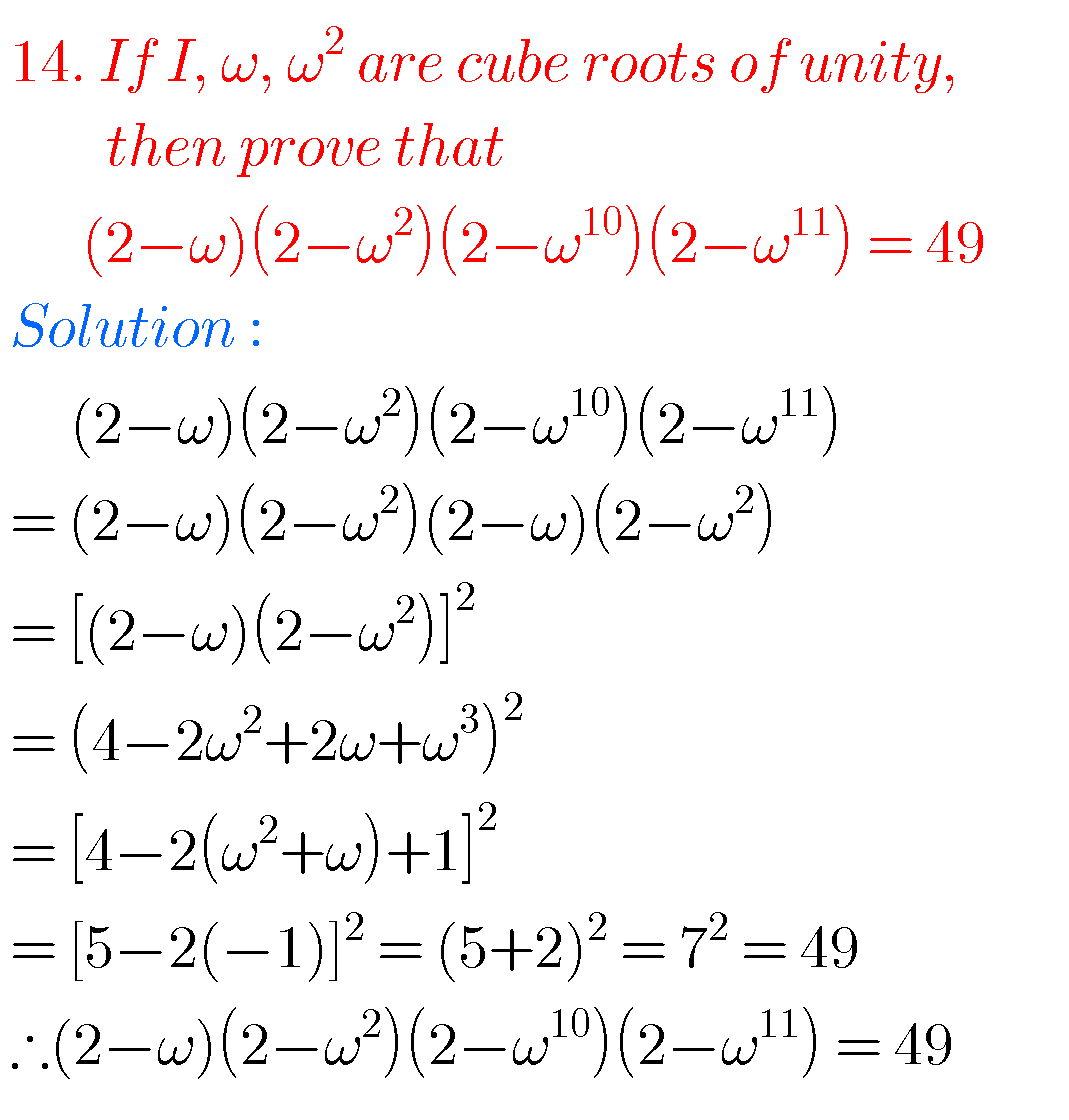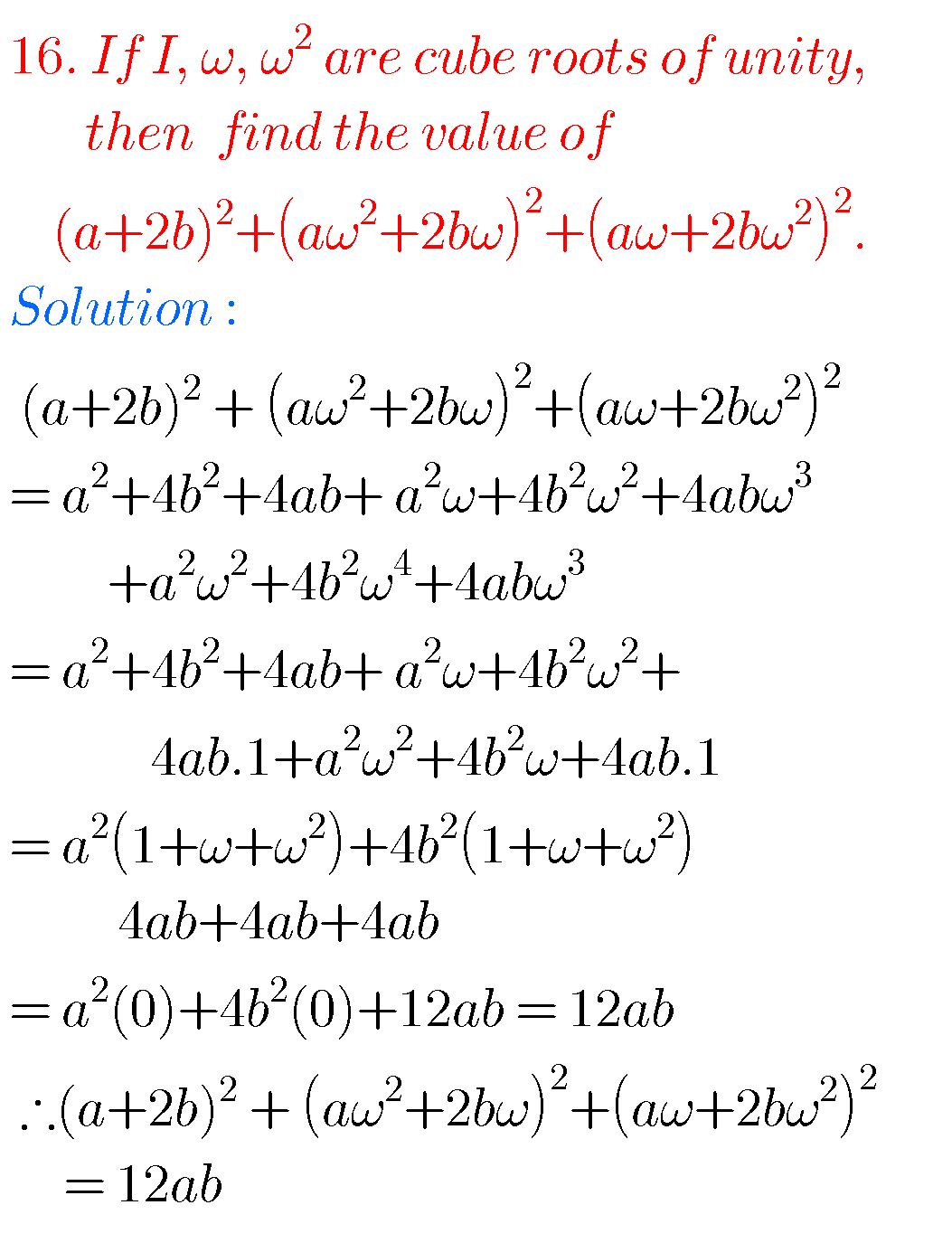## Solutions for inter maths 2a chapter 2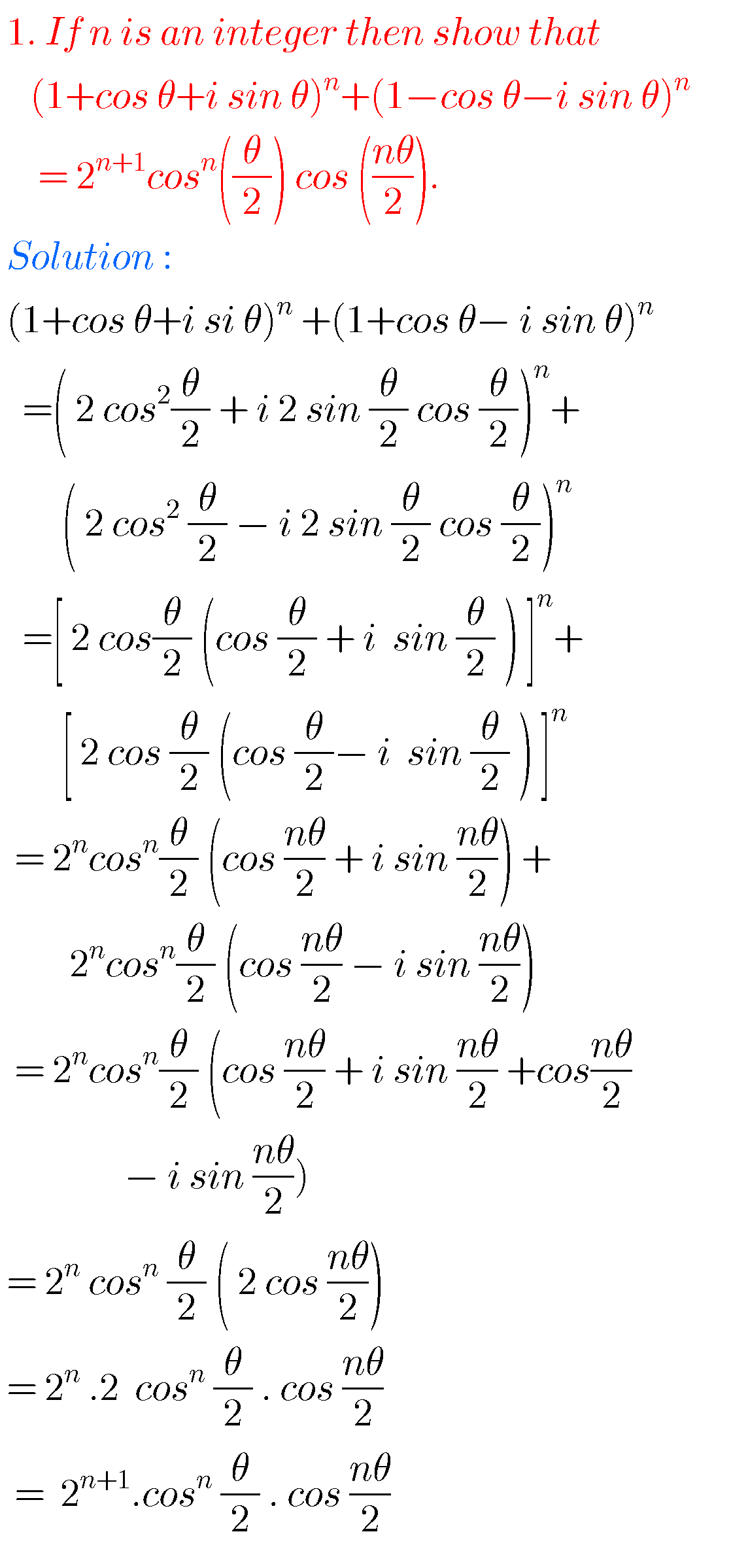###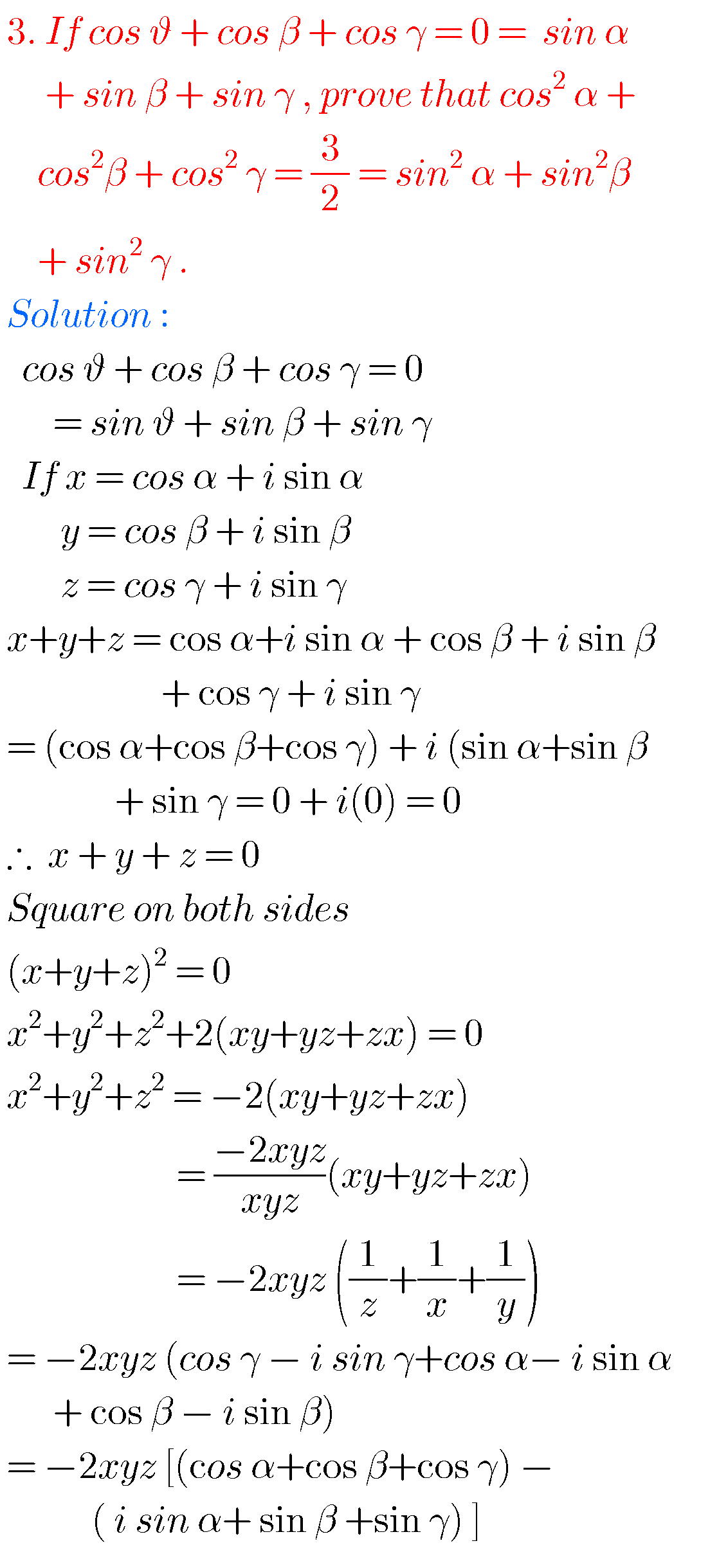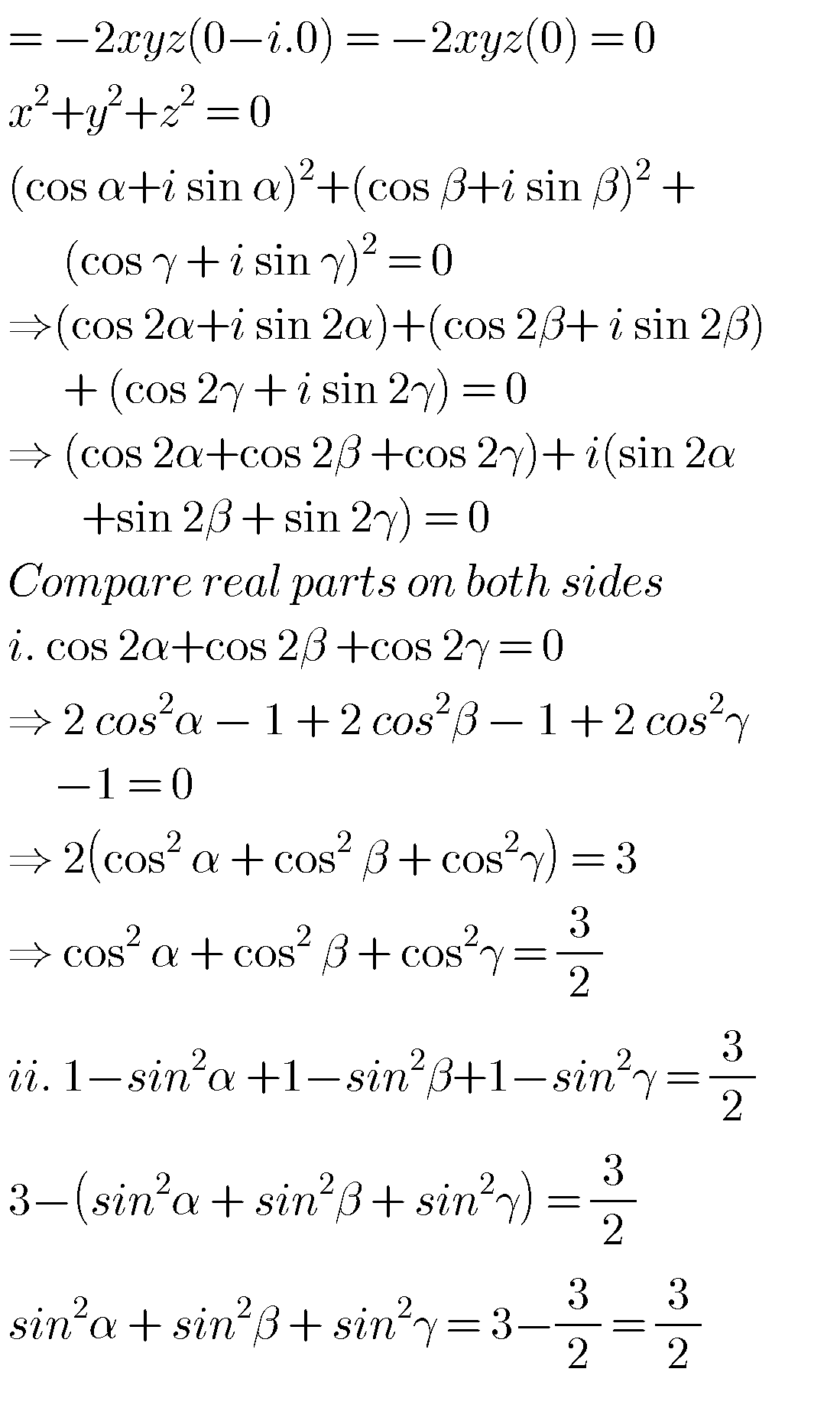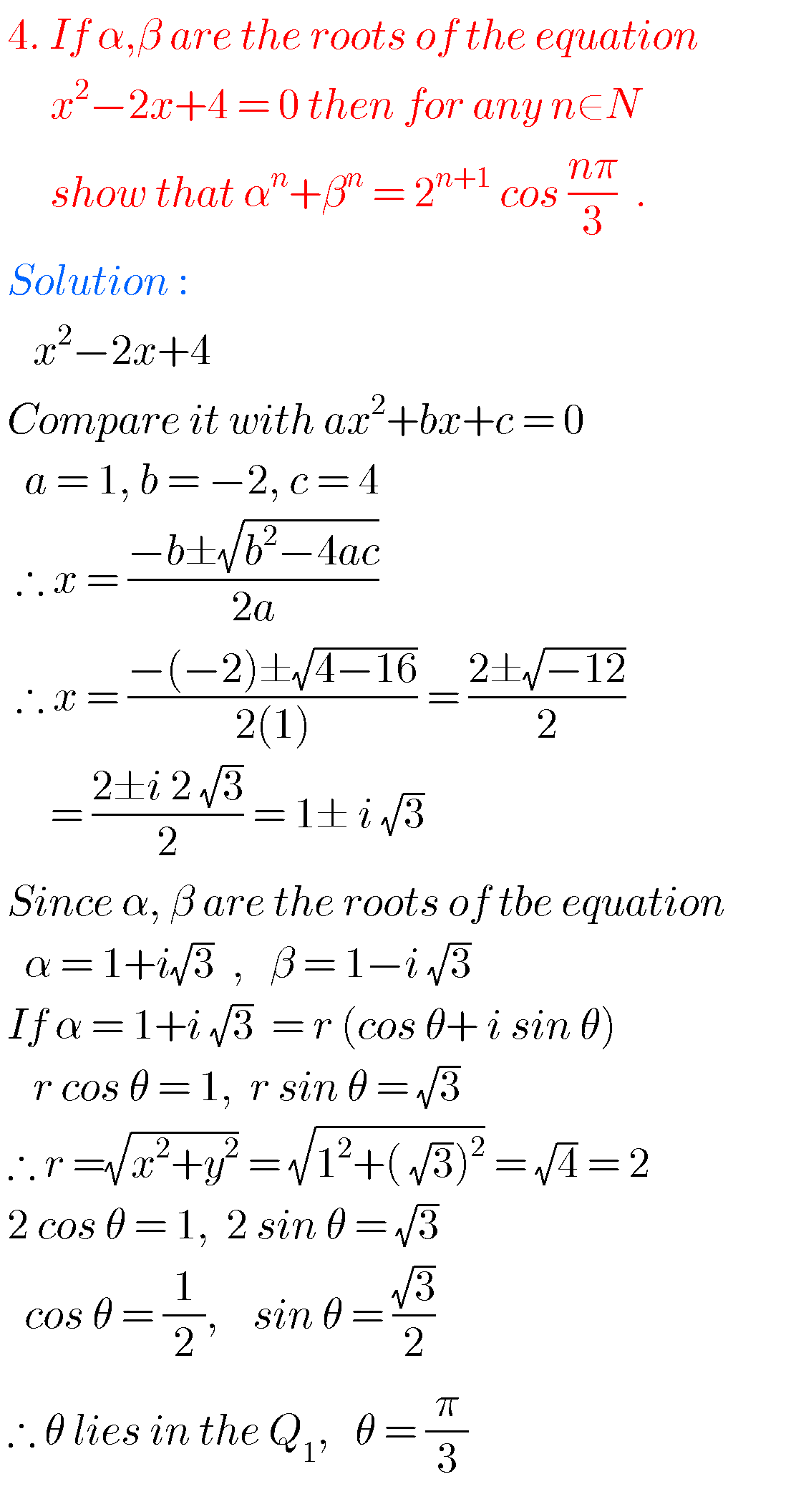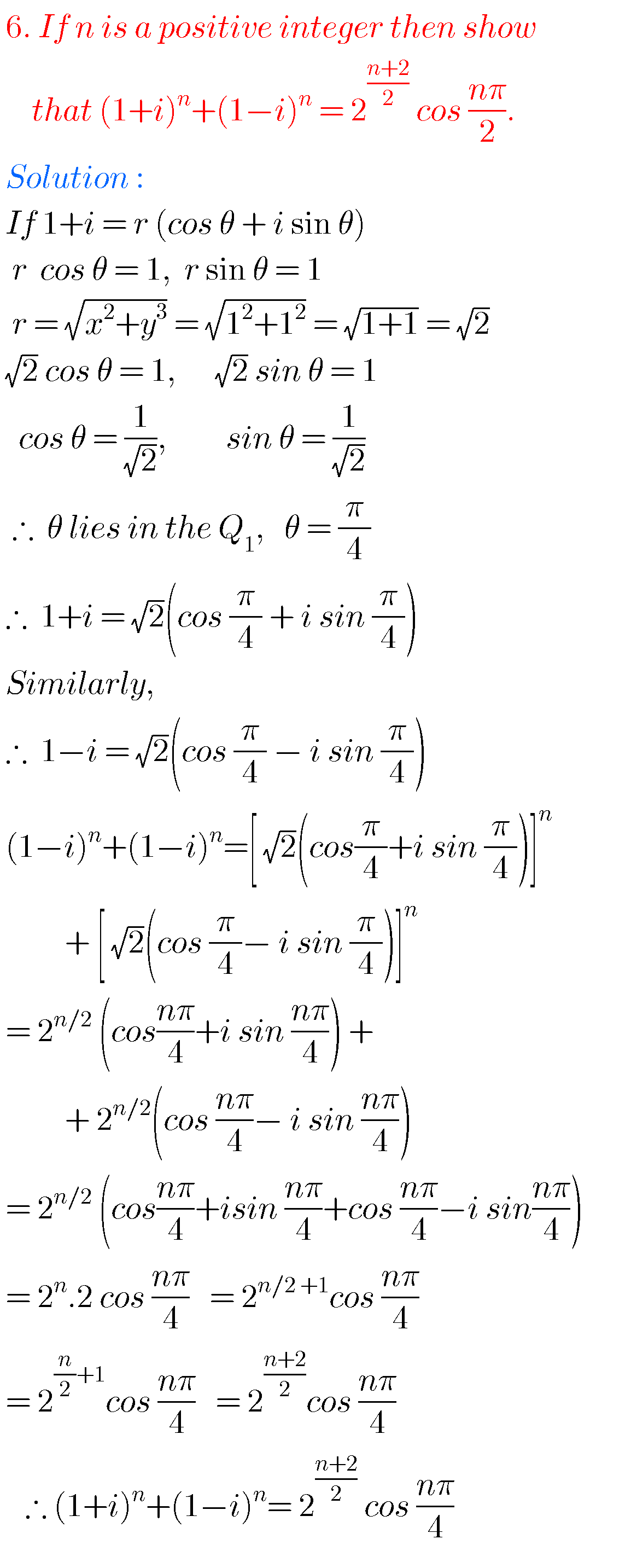###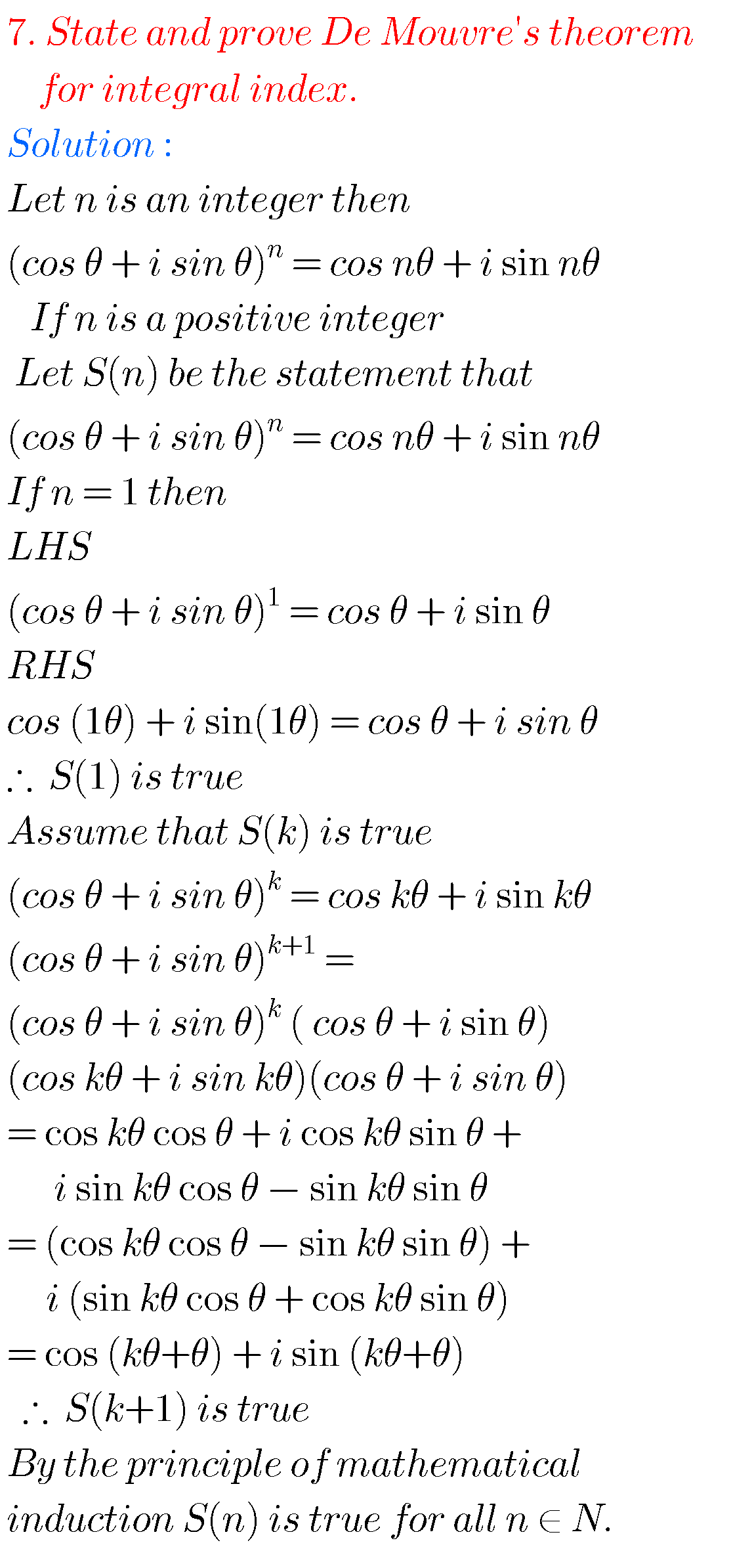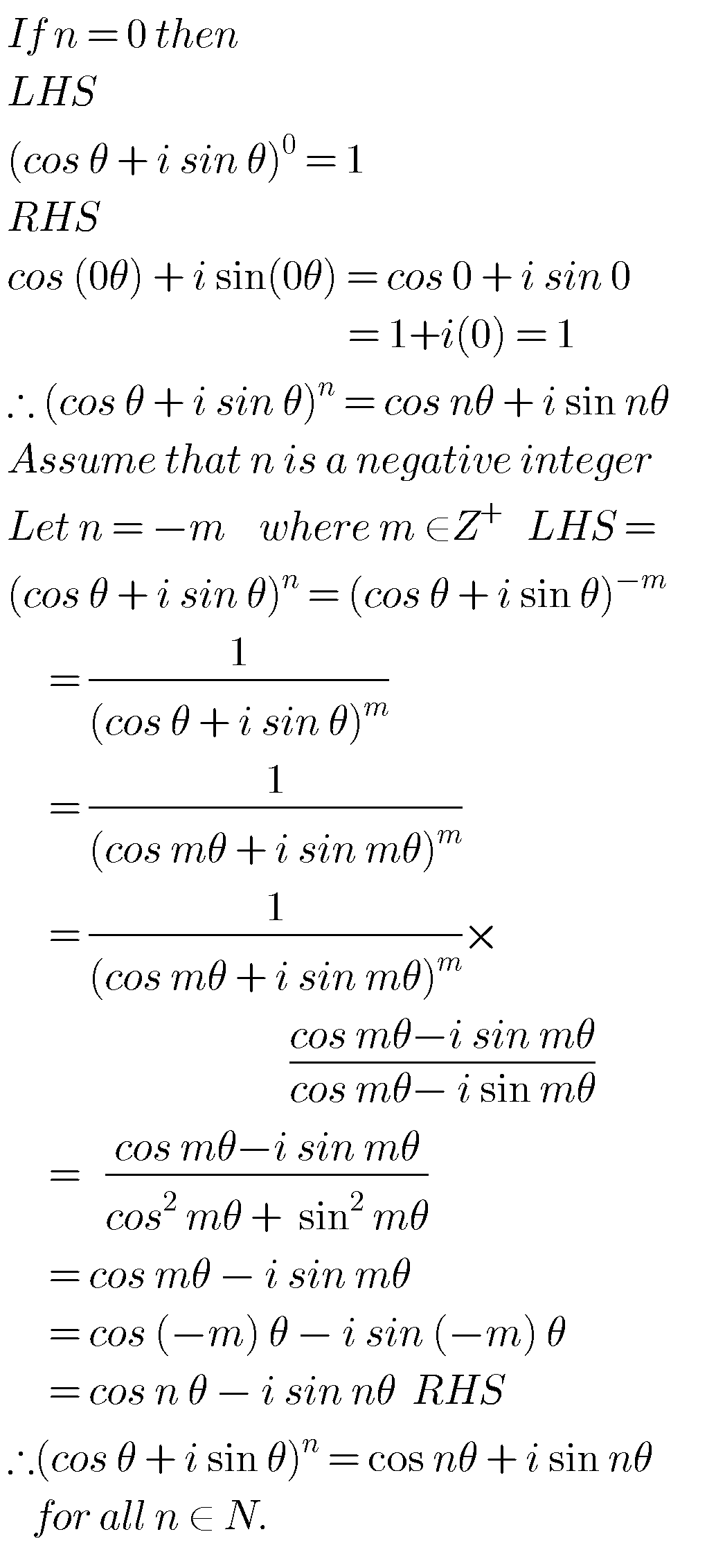###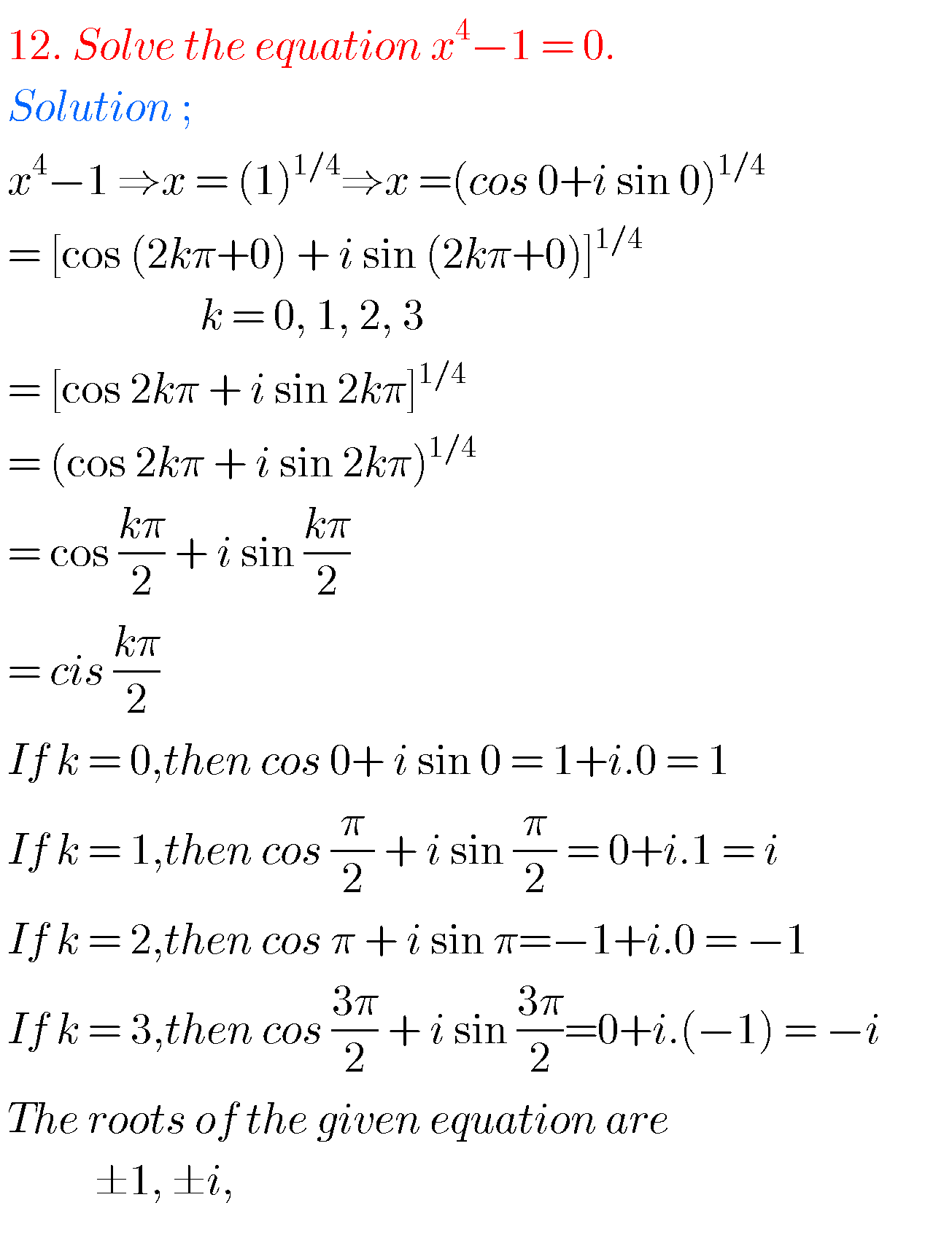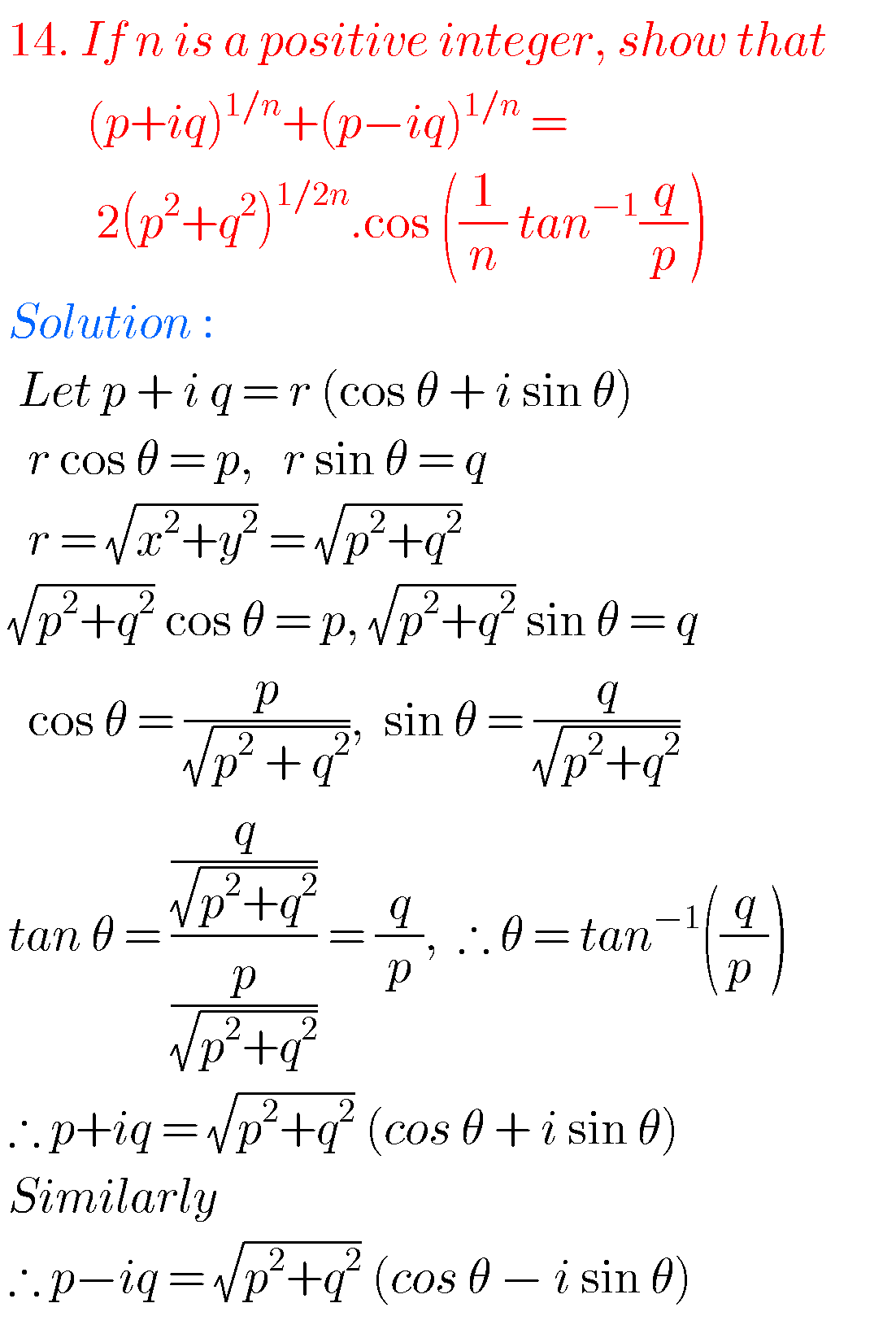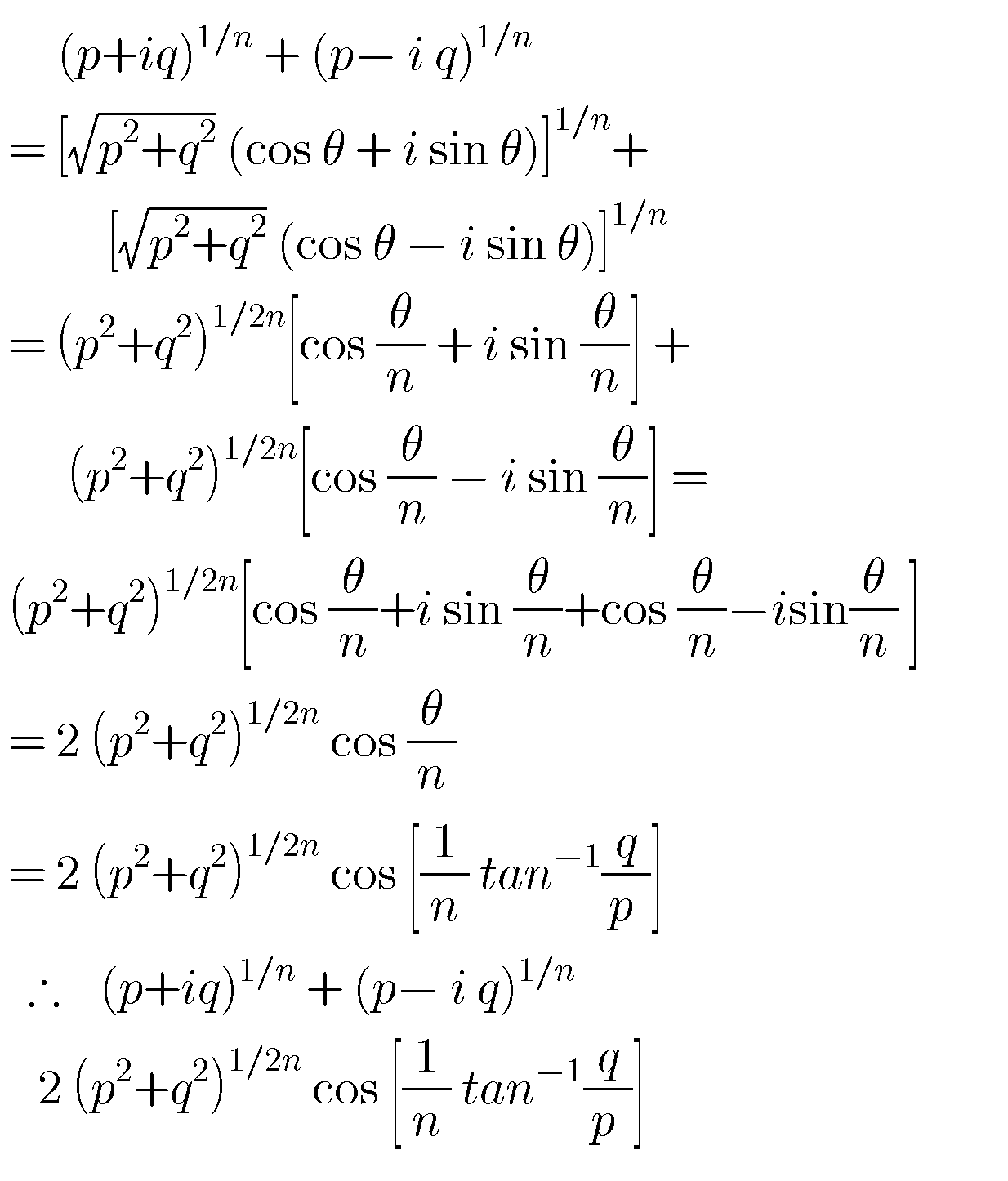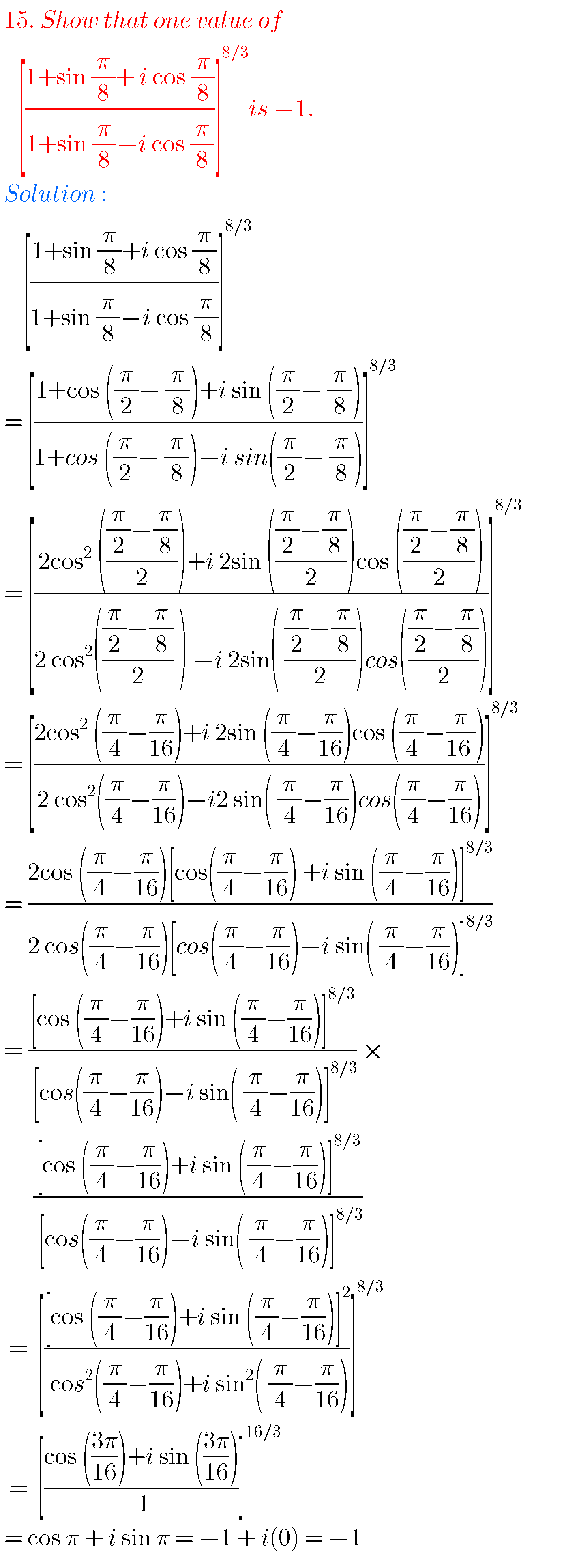### Mathematics solutions for de moivre’s theorem inter IIA

Note : Observe the solutions and try them in your own methods.

10 class maths real numbers solutions

Ncert solutions for class 8 linear equations

Ncert solutions for class 6 integers